# Methods To Check The Performance Of Regression Models

The model-building process is the core part of Machine Learning. There is much research going on in this area to build a more accurate model, and when people publish the results, they try to compare the performance of their models based on which we can decide Which one is more accurate and robust? And, Which model architecture should we pick?

Whenever we say that we have built any model, the first question that comes to us is, “What is the accuracy of your model?”. Unfortunately, we have a continuous target variable in a regression problem, and predicting the accurate value is impossible. Hence we can not measure accuracy here. However, to compare the performance of the regression models, there are some defined metrics based on which we can decide which model is performing better. So let’s list down some most common metrics.

1. Mean Absolute Error (MAE)
2. Mean Absolute Percentage Error (MAPE)
3. Mean Squared Error (MSE)
4. Root Mean Squared Error (RMSE)
5. R-Squared ()

#### Mean Absolute Error: MAE

When we perform the inference from our built model, it produces some output. As this output is continuous, we can always calculate the difference between the predicted value and the actual value. Let’s say Ŷi is the predicted value, and Yi is the actual value. So, we can define error in prediction as

Error = Ŷi-Yi or Error = Yi-Ŷi, or simply we can write, Error = |Yi-Ŷi|.

Now, as we know that the target variable Y is continuous, and let’s say we have N samples from the continuous domain, the total error would be the sum of errors over all those samples, i.e., Total error =|Yi-Ŷi|.*

As the name suggests, we need to take the mean of this error, which can be calculated by dividing the total error by the number of samples. I.e.,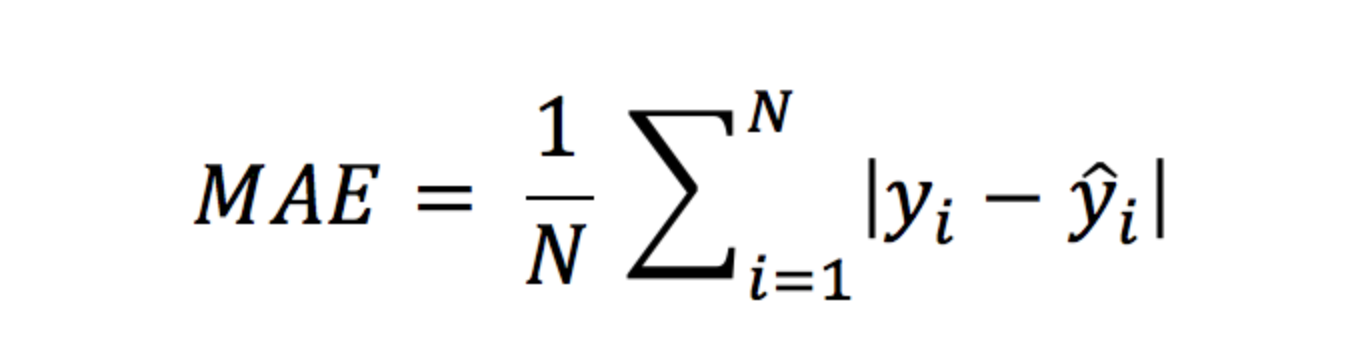``````from sklearn.metrics import mean_absolute_error
print("MAE = ",mean_absolute_error(y_true, y_pred))
``````

#### Mean Absolute Percentage Error: MAPE

In different research works, it can be observed that when the target variable feature has a single dimension, some research performs Normalization over that target feature, and some don't. For example, suppose our target variable ranges in [0–100], and one method normalized this feature and brought it in the range of [0,1]. But in such a scenario, for the same model, the value of MAE would vary. The error in the unnormalized case would be higher than the error in the normalized case.
To tackle this, we can define our error in terms of percentage variation from the actual values. In the equation below, Yi is the actual value, and Ŷi is the predicted value, and N is the total number of samples.``````from sklearn.metrics import mean_absolute_percentage_error
print("MAPE = ",mean_absolute_percentage_error(y_true, y_pred))
``````

#### Mean Squared Error: MSE

Mean Square Error is an absolute measure of the goodness for the fit. It is similar to the mean absolute error, but the error here is calculated as Error = |Yi-Ŷi|². And similar to the MAE, when this squared error would be calculated for N samples, Total error =|Yi-Ŷi|².* And to represent this value as a Mean Squared Error,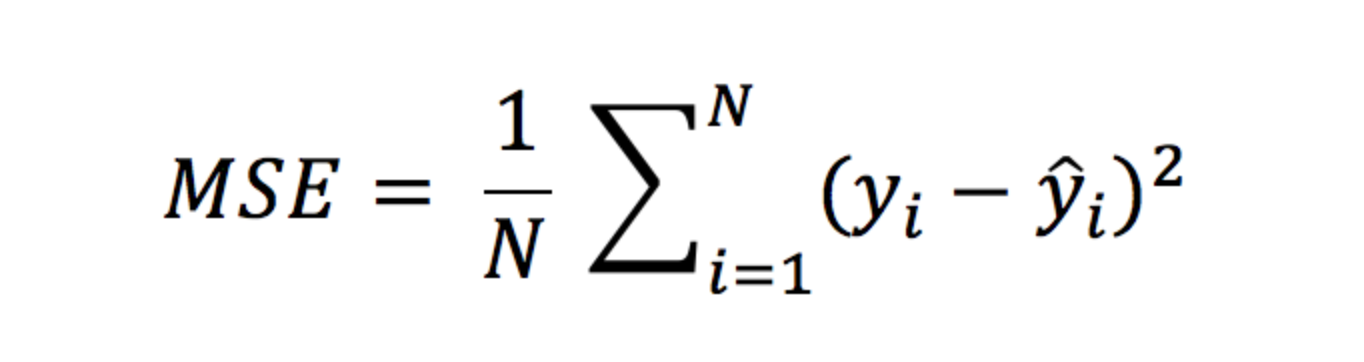``````from sklearn.metrics import mean_squared_error
print("MSE = ",mean_squared_error(y_true, y_pred))
``````

#### Root Mean Squared Error: RMSE

RMSE is the most famous evaluation metric for the regression model. The overall calculation of RMSE is similar to MSE; just the final value is square-rooted as we calculated the square of errors in MSE.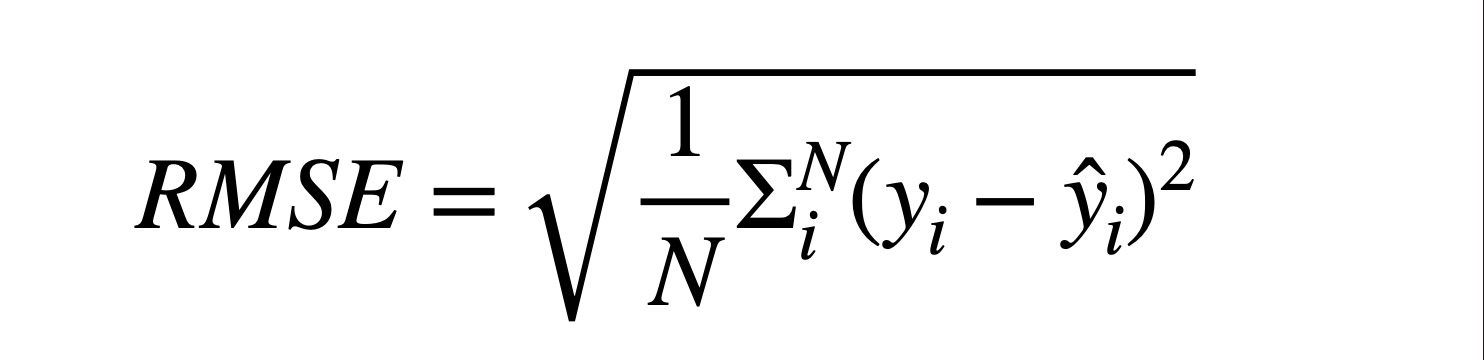``````from sklearn.metrics import mean_squared_error
import nunpy as np
print("RMSE = ",np.sqrt(mean_squared_error(y_true, y_pred)))
``````

#### R-Squared: R²

It is also known as the Coefficient of Determination. Correlation between independent and dependant variables explains the strength of their relationship. In contrast, R-squared explains to what extent the variance of one variable explains the variance of the second variable. R-Square can be calculated using the equation below in which y̅i is the mean of Ŷi is the predicted value.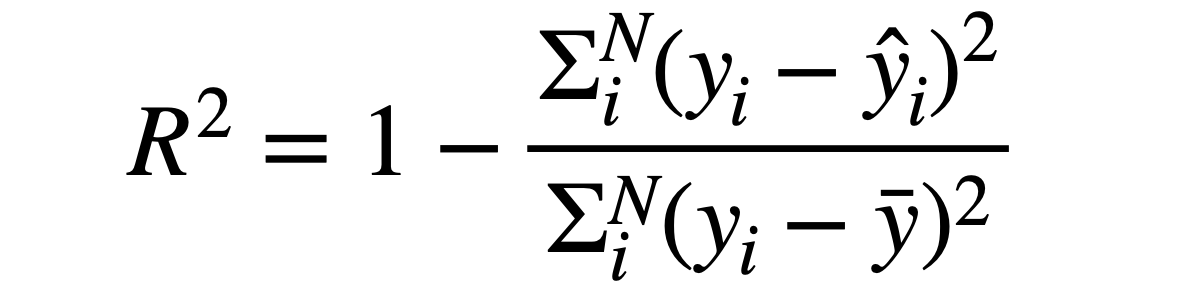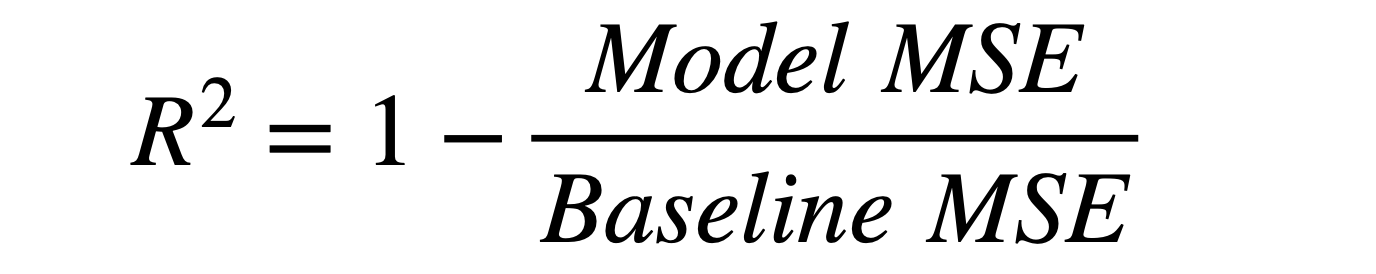``````from sklearn.metrics import r2_score
print("R_Squared = ",r2_score(y_true, y_pred))
``````

In theories, the R_squared value will always lie in the range of [0,1], but in practice, the values lie in the range of (-∞, 1]. The reason behind negative R² can be,

1. Model is not learning the trend that is present in the train data.
2. Too little data has been used to evaluate the model when compared to train data.
3. Too many outliers are present in the dataset.

R² is a good measure and is widely used in industry to measure the performance of models. But there are serious problems that can misguide machine learning engineers and researchers. If there are too many independent variables, the model can overfit, and R² would be really high. But on the test data, it will perform poorly.

To tackle the problems of R², researchers formed a new metric that is considered the improvement in R² and known as adjusted R². In the equation below, N is the total number of data samples, and k is the number of independent variables in the data.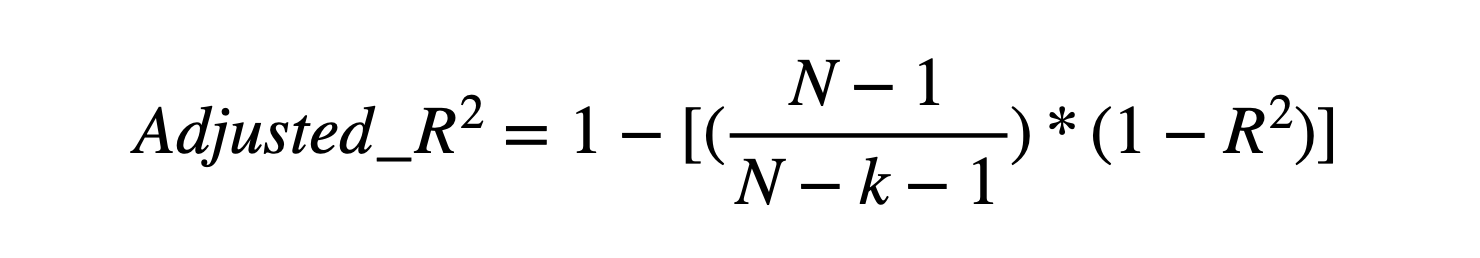``````from sklearn.metrics import r2_score
r_sqr = r2_score(y_true, y_pred)
N = len(y_true)
k = # of independent variable in input features.
``````

The value of Adjusted R-Squared will always be lesser than the traditional R-Squared value.

#### Possible Interview Questions

1. What are the standard methods used for evaluating our machine learning model’s performance?
2. What is MAPE, and why is it more helpful?
3. What is the range of R² values? What can be its limitations?
4. What people prefer R² rather than adjusted R²?

#### Recommendation and Conclusion

Industries and Research papers are more inclined towards RMSE or MSE values, so we must compare our results with these parameters. Additionally, there is a slight inclination towards R-Squared values as well as it can be directly correlated with the accuracy. Adjusted R² is the only parameter considering the overfitting problem. But due to the dependency on several independent features, there is no direct library available in most frameworks to calculate it. We hope you enjoyed the article.

#### Enjoy Learning! Enjoy Thinking! Enjoy Algorithms!

Get well-designed application and interview centirc content on ds-algorithms, machine learning, system design and oops. Content will be delivered weekly.